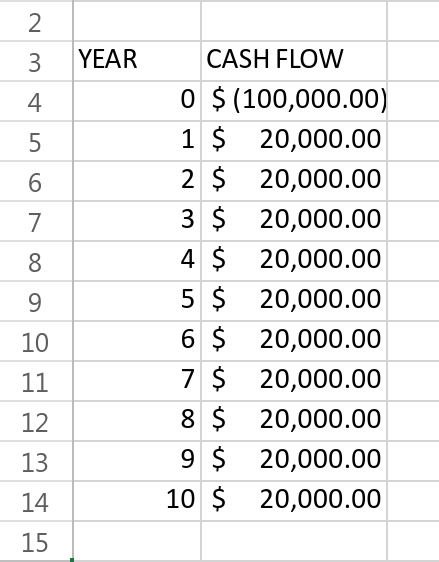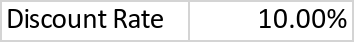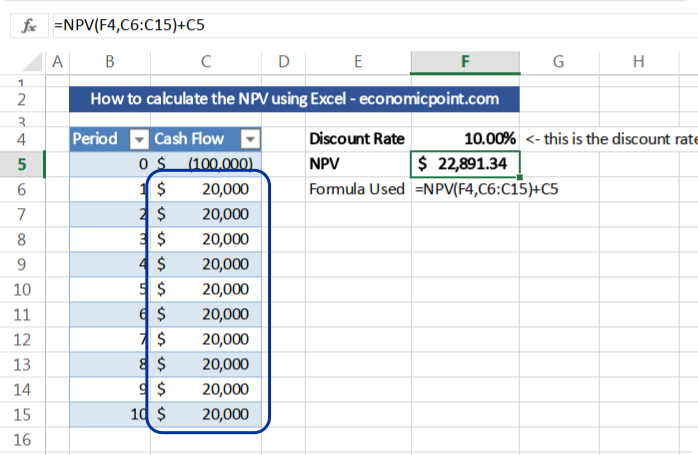# How to Calculate the NPV in Excel?

This article teaches you how to calculate the NPV (Net Present Value) using Excel. The Excel function to calculate the NPV is “NPV”.

The NPV, or Net Present Value, is the present value, or actual value, of a future flow of funds. The present value of a future cash flow is the current worth of it. To know the current value, you must use a discount rate. The NPV includes not only the positive cash flows, or inflows, but also all expenditures, including the initial investment.

If the NPV is positive, it means that the actual value of all incomes is higher than the actual value of all expenditures, and the investment is desirable, because it adds more value than the best alternative.

The cash flows are discounted using a discount rate that corresponds to the best alternative investment.

STEP 1: Know your Cash Flow

The first step to calculate the NPV using Excel is to input all the cash flows of the investment project. Usually, the flows are calculated annually, but you can use months also. Take into account that the discount rate period must correspond to the cash flows period. For example, if you use annual cash flows, the discount rate must be annual.

The cash flows are usually estimated using a model.STEP 2: Know your Discount Rate

Select a cell and enter your discount rate. For example, if your discount rate is 10%, you should enter 0.1

Remember that the discount rate is the rate of return of your best alternative investment with similar risk to the current project.STEP 3: Enter the NPV Excel Formula

The NPV formula requires 2 arguments:

1. The discount rate: cell E1 in our example
2. The cash flow range: range B5:B14 in our example.

Please take into account that the Excel NPV formula starts with the first period, and the initial investment occurs in period “0”. So, we must subtract the initial investment from the previous calculation. `Note: some websites are wrong, they include the initial investment into the NPV formula. This leads to a minor difference, because Excel assumes the first period is one year in advance.`

## Excel Formula:

`=NPV(E3,B5:B14)+B4`

Where:
E3: cell containing the discount rate.
B5:B14: range containing the future cash flows
B4: cell containing the initial investment (in this example, the initial investment is expressed in negative values, that is why we add it)

Press Enter and Excel will calculate the NPV.